# EHP spectral sequence

(diff) ← Older revision | Latest revision (diff) | Newer revision → (diff)

An inductive procedure to calculate the homotopy groups of spheres (cf. Spheres, homotopy groups of the). It has the attractive feature that the input for the calculation is always a result of an earlier calculation once one inputs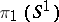. It has been used to calculate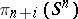for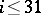. The last of these calculations are contained in [a3] and complete references can be found there. It is constructed by splicing together fibrations discovered by I.M. James [a2] at the prime numberand at odd prime numbers by H. Toda [a4].

In this paragraph the case of the prime numberis discussed, and all spaces are-local and all groups should be considered as completed at. James showed that there is a-local fibration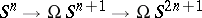. This gives rise to long exact sequences (cf. Exact sequence)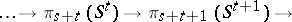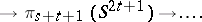The first mapping is usually called, the secondand the connecting homomorphism. The EHP spectral sequence is obtained by defining the filtration of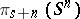by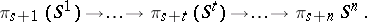This gives rise to a spectral sequence with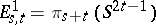and converging to. There are several important features of this spectral sequence. First, note that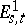is itself a result of another calculation using this spectral sequence for smaller values of. The value ofis called the stem and the filtration parametergives the sphere of origin of a class. The name a homotopy class receives from this spectral sequence is called the Hopf invariant of the class. Lettinggo to infinity gives a spectral sequence which calculates the stable homotopy groups (cf. Stable homotopy group). The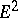term does not have a special name, but there is the following result. For all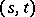,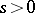, and odd,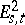is an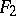vector space [a1]. The differential is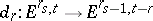.

For odd prime numbers, the situation is slightly more complicated. In this paragraph all spaces are-local for a fixed odd prime numberand all groups are completed at. First there is the result of James,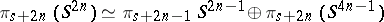. This reduces the calculation for even-dimensional spheres to that of odd-dimensional spheres. In order to get Toda's version of the EHP sequence, one introduces a modified even sphere. Let, the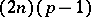-skeleton of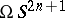. Then Toda showed that the following are-local fibrations: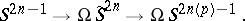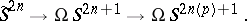As in the primecase, one can fit the long exact sequences in homotopy together to get a spectral sequence. It is associated to the filtration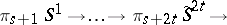As before, one can use Toda's sequences to identify the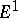-term: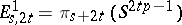and. This spectral sequence converges to the-local homotopy of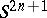. As before, the input is the result of an earlier calculation. There is also the classical result thatis anvector space. The parameterrefers to the stem and the parameterrefers to the sphere of origin. Here, this refers to the odd sphere or the modified even sphere of origin. The name a class gets in this spectral sequence is also called the Hopf invariant.

How to Cite This Entry:
EHP spectral sequence. Encyclopedia of Mathematics. URL: http://encyclopediaofmath.org/index.php?title=EHP_spectral_sequence&oldid=18009
This article was adapted from an original article by M. Mahowald (originator), which appeared in Encyclopedia of Mathematics - ISBN 1402006098. See original article